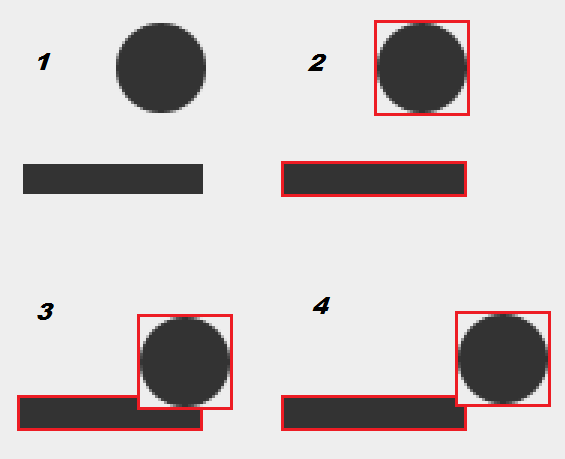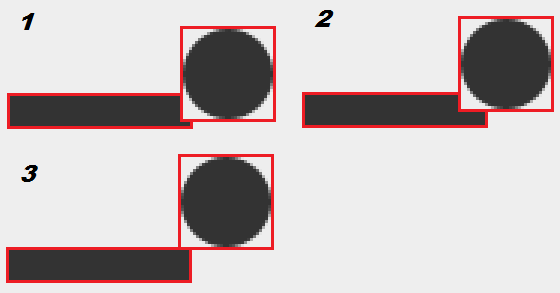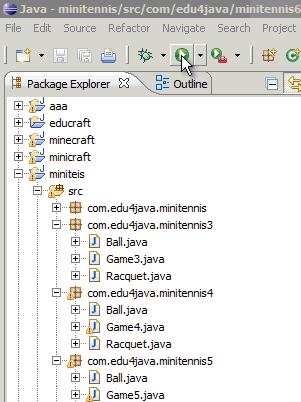# Collision detection

In this tutorial we will learn how to detect when a sprite collides with another. In our game we will make the ball bounce on the racquet. We will also make the game finish if the ball gets to the lower border of the canvas, showing a popup window with the classic message "Game Over".

## Game Over

Below we can see our class Game, which is exactly the same as the previous one, with the only difference that this one has a gameOver() method;

```package com.edu4java.minitennis6;

import java.awt.Graphics;
import java.awt.Graphics2D;
import java.awt.RenderingHints;
import java.awt.event.KeyEvent;
import java.awt.event.KeyListener;
import javax.swing.JFrame;
import javax.swing.JOptionPane;
import javax.swing.JPanel;

@SuppressWarnings("serial")
public class Game extends JPanel {

Ball ball = new Ball(this);
Racquet racquet = new Racquet(this);

public Game() {
@Override
public void keyTyped(KeyEvent e) {
}

@Override
public void keyReleased(KeyEvent e) {
racquet.keyReleased(e);
}

@Override
public void keyPressed(KeyEvent e) {
racquet.keyPressed(e);
}
});
setFocusable(true);
}

private void move() {
ball.move();
racquet.move();
}

@Override
public void paint(Graphics g) {
super.paint(g);
Graphics2D g2d = (Graphics2D) g;
g2d.setRenderingHint(RenderingHints.KEY_ANTIALIASING,
RenderingHints.VALUE_ANTIALIAS_ON);
ball.paint(g2d);
racquet.paint(g2d);
}

public void gameOver() {
JOptionPane.showMessageDialog(this, "Game Over", "Game Over", JOptionPane.YES_NO_OPTION);
System.exit(ABORT);
}

public static void main(String[] args) throws InterruptedException {
JFrame frame = new JFrame("Mini Tennis");
Game game = new Game();
frame.setSize(300, 400);
frame.setVisible(true);
frame.setDefaultCloseOperation(JFrame.EXIT_ON_CLOSE);

while (true) {
game.move();
game.repaint();
}
}
}```

The gameOver() method launches a popup using JOptionPane.showMessageDialog with the message "Game Over" and an "Accept" button. After the popup, System.exit(ABORT) makes the program finish. The gameOver() method is public, because it will be called from the sprite "Ball" when it detects that it has got to the lower border of the canvas.

## Sprite collision

To detect the collision between the ball and the racquet we will use rectangles. In the case of the ball we will use a square around the ball as you can see in the figure 2.The class java.awt.Rectangle has an intersects method(Rectangle r) which returns true when two rectangles occupy the same space, like in the case of the figure 3 or 4. This method is not quite exact, because as you can see in the figure 4, the ball doesn´t touch the racquet, but for our example it it more than enough.

Below we can see the Racquet class, with the only difference that we have added a getBounds() method, which returns a rectangle type of object, indicating the position of the racquet. This method will be used by the sprite "Ball", to know the position of the racquet and in this way to detect the collision.

```package com.edu4java.minitennis6;

import java.awt.Graphics2D;
import java.awt.Rectangle;
import java.awt.event.KeyEvent;

public class Racquet {
private static final int Y = 330;
private static final int WIDTH = 60;
private static final int HEIGHT = 10;
int x = 0;
int xa = 0;
private Game game;

public Racquet(Game game) {
this.game = game;
}

public void move() {
if (x + xa > 0 && x + xa < game.getWidth() - WIDTH)
x = x + xa;
}

public void paint(Graphics2D g) {
g.fillRect(x, Y, WIDTH, HEIGHT);
}

public void keyReleased(KeyEvent e) {
xa = 0;
}

public void keyPressed(KeyEvent e) {
if (e.getKeyCode() == KeyEvent.VK_LEFT)
xa = -1;
if (e.getKeyCode() == KeyEvent.VK_RIGHT)
xa = 1;
}

public Rectangle getBounds() {
return new Rectangle(x, Y, WIDTH, HEIGHT);
}

public int getTopY() {
return Y;
}
}```

Another small change is the inclusion of constants:

```	private static final int Y = 330;
private static final int WIDTH = 60;
private static final int HEIGH = 20;```

As we said before, the value of the "y" position, was fixed to 330. This value is used both in the paint method as in getBounds. When we create a constant, the good thing is that if we want to change the value, we only have to change it in one place. In this way we avoid the possible error of changing it in one place and not changing it in another.

The way of defining a constant is declaring a "static final" property and writing it in upper case. The compiler allows us to use lower case, but the standar says we use upper case for the constants.

Lastly, the Ball class:

```package com.edu4java.minitennis6;

import java.awt.Graphics2D;
import java.awt.Rectangle;

public class Ball {
private static final int DIAMETER = 30;
int x = 0;
int y = 0;
int xa = 1;
int ya = 1;
private Game game;

public Ball(Game game) {
this.game= game;
}

void move() {
if (x + xa < 0)
xa = 1;
if (x + xa > game.getWidth() - DIAMETER)
xa = -1;
if (y + ya < 0)
ya = 1;
if (y + ya > game.getHeight() - DIAMETER)
game.gameOver();
if (collision()){
ya = -1;
y = game.racquet.getTopY() - DIAMETER;
}
x = x + xa;
y = y + ya;
}

private boolean collision() {
return game.racquet.getBounds().intersects(getBounds());
}

public void paint(Graphics2D g) {
g.fillOval(x, y, DIAMETER, DIAMETER);
}

public Rectangle getBounds() {
return new Rectangle(x, y, DIAMETER, DIAMETER);
}
}```

In a similar way, we have included the getBounds() method and the DIAMETER constant to the class "Racquet".

More interesting is the inclusion of the new method called collision() which returns true, if the rectangle occupied by the racquet "game.racquet.getBounds()" intersects with the rectangle occupied by the ball "getBounds()".

```	private boolean collision() {
return game.racquet.getBounds().intersects(getBounds());
}```

If the collision takes place, we will change the direction and the position of the ball. If the collision occurs on the side (figure 1), the ball could be several pixels below the upper side of the racquet. In the following game loop, even if the ball moved upwards (figure 2), it could still be in collision with the racquet.To avoid this, we will place the ball on top of the racquet (figure 3) using:

` 	y = game.racquet.getTopY() - DIAMETER;`

The "Racquet" getTopY() method gives us the position in the "y" axis of the upper part of the racquet, and if we discount the DIAMETER, we obtain the exact position where to put the ball so that it is on top of the ball.

Lastly, it is the move() method of the "Ball" class which uses the new methods collision() and gameOver() of the "Game" class. The bounce when it gets to the lower border is replaced by a call to game.gameOver().

```		if (y + ya > game.getHeight() - DIAMETER)
game.gameOver();
```

And including a new conditional using the collision() method, we get the ball to bounce upwards if it collides with the racquet:

```		if (collision())
ya = -1;```

If we run the example we can see: